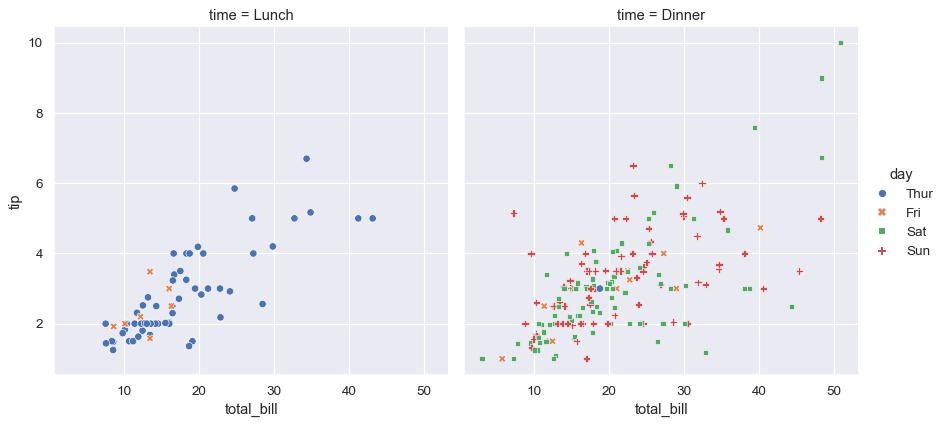# seaborn.scatterplot#

seaborn.scatterplot(data=None, *, x=None, y=None, hue=None, size=None, style=None, palette=None, hue_order=None, hue_norm=None, sizes=None, size_order=None, size_norm=None, markers=True, style_order=None, legend='auto', ax=None, **kwargs)#

Draw a scatter plot with possibility of several semantic groupings.

The relationship between `x` and `y` can be shown for different subsets of the data using the `hue`, `size`, and `style` parameters. These parameters control what visual semantics are used to identify the different subsets. It is possible to show up to three dimensions independently by using all three semantic types, but this style of plot can be hard to interpret and is often ineffective. Using redundant semantics (i.e. both `hue` and `style` for the same variable) can be helpful for making graphics more accessible.

The default treatment of the `hue` (and to a lesser extent, `size`) semantic, if present, depends on whether the variable is inferred to represent “numeric” or “categorical” data. In particular, numeric variables are represented with a sequential colormap by default, and the legend entries show regular “ticks” with values that may or may not exist in the data. This behavior can be controlled through various parameters, as described and illustrated below.

Parameters:
data`pandas.DataFrame`, `numpy.ndarray`, mapping, or sequence

Input data structure. Either a long-form collection of vectors that can be assigned to named variables or a wide-form dataset that will be internally reshaped.

x, yvectors or keys in `data`

Variables that specify positions on the x and y axes.

huevector or key in `data`

Grouping variable that will produce points with different colors. Can be either categorical or numeric, although color mapping will behave differently in latter case.

sizevector or key in `data`

Grouping variable that will produce points with different sizes. Can be either categorical or numeric, although size mapping will behave differently in latter case.

stylevector or key in `data`

Grouping variable that will produce points with different markers. Can have a numeric dtype but will always be treated as categorical.

palettestring, list, dict, or `matplotlib.colors.Colormap`

Method for choosing the colors to use when mapping the `hue` semantic. String values are passed to `color_palette()`. List or dict values imply categorical mapping, while a colormap object implies numeric mapping.

hue_ordervector of strings

Specify the order of processing and plotting for categorical levels of the `hue` semantic.

hue_norm

Either a pair of values that set the normalization range in data units or an object that will map from data units into a [0, 1] interval. Usage implies numeric mapping.

sizeslist, dict, or tuple

An object that determines how sizes are chosen when `size` is used. List or dict arguments should provide a size for each unique data value, which forces a categorical interpretation. The argument may also be a min, max tuple.

size_orderlist

Specified order for appearance of the `size` variable levels, otherwise they are determined from the data. Not relevant when the `size` variable is numeric.

size_normtuple or Normalize object

Normalization in data units for scaling plot objects when the `size` variable is numeric.

markersboolean, list, or dictionary

Object determining how to draw the markers for different levels of the `style` variable. Setting to `True` will use default markers, or you can pass a list of markers or a dictionary mapping levels of the `style` variable to markers. Setting to `False` will draw marker-less lines. Markers are specified as in matplotlib.

style_orderlist

Specified order for appearance of the `style` variable levels otherwise they are determined from the data. Not relevant when the `style` variable is numeric.

legend“auto”, “brief”, “full”, or False

How to draw the legend. If “brief”, numeric `hue` and `size` variables will be represented with a sample of evenly spaced values. If “full”, every group will get an entry in the legend. If “auto”, choose between brief or full representation based on number of levels. If `False`, no legend data is added and no legend is drawn.

ax`matplotlib.axes.Axes`

Pre-existing axes for the plot. Otherwise, call `matplotlib.pyplot.gca()` internally.

kwargskey, value mappings

Other keyword arguments are passed down to `matplotlib.axes.Axes.scatter()`.

Returns:
`matplotlib.axes.Axes`

The matplotlib axes containing the plot.

`lineplot`

Plot data using lines.

`stripplot`

Plot a categorical scatter with jitter.

`swarmplot`

Plot a categorical scatter with non-overlapping points.

Examples

These examples will use the “tips” dataset, which has a mixture of numeric and categorical variables:

```tips = sns.load_dataset("tips")
```
total_bill tip sex smoker day time size
0 16.99 1.01 Female No Sun Dinner 2
1 10.34 1.66 Male No Sun Dinner 3
2 21.01 3.50 Male No Sun Dinner 3
3 23.68 3.31 Male No Sun Dinner 2
4 24.59 3.61 Female No Sun Dinner 4

Passing long-form data and assigning `x` and `y` will draw a scatter plot between two variables:

```sns.scatterplot(data=tips, x="total_bill", y="tip")
```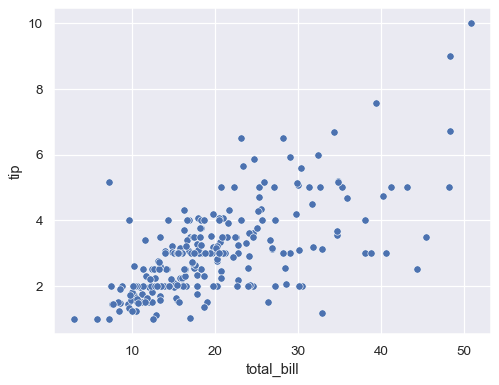Assigning a variable to `hue` will map its levels to the color of the points:

```sns.scatterplot(data=tips, x="total_bill", y="tip", hue="time")
```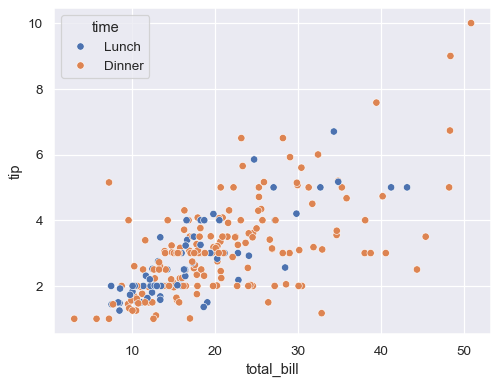Assigning the same variable to `style` will also vary the markers and create a more accessible plot:

```sns.scatterplot(data=tips, x="total_bill", y="tip", hue="time", style="time")
```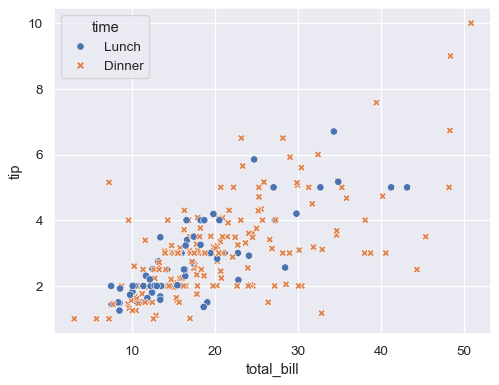Assigning `hue` and `style` to different variables will vary colors and markers independently:

```sns.scatterplot(data=tips, x="total_bill", y="tip", hue="day", style="time")
```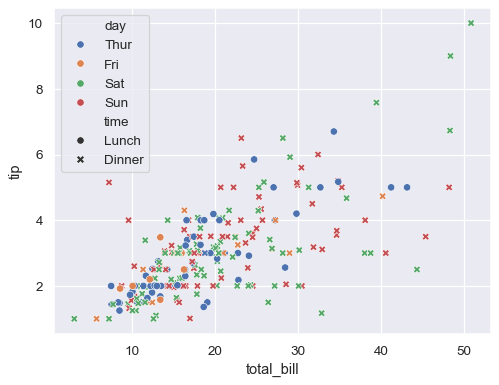If the variable assigned to `hue` is numeric, the semantic mapping will be quantitative and use a different default palette:

```sns.scatterplot(data=tips, x="total_bill", y="tip", hue="size")
```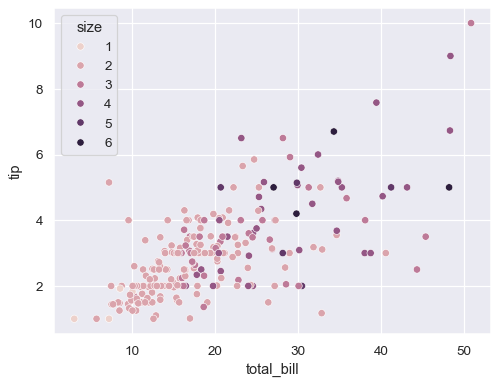Pass the name of a categorical palette or explicit colors (as a Python list of dictionary) to force categorical mapping of the `hue` variable:

```sns.scatterplot(data=tips, x="total_bill", y="tip", hue="size", palette="deep")
```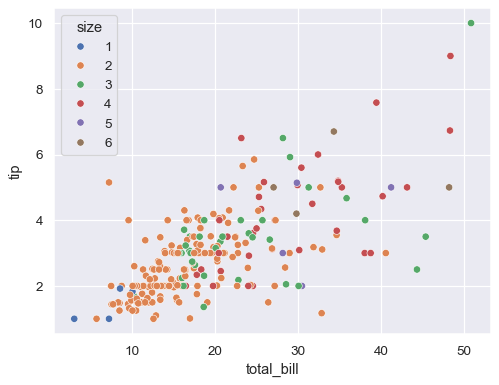If there are a large number of unique numeric values, the legend will show a representative, evenly-spaced set:

```tip_rate = tips.eval("tip / total_bill").rename("tip_rate")
sns.scatterplot(data=tips, x="total_bill", y="tip", hue=tip_rate)
```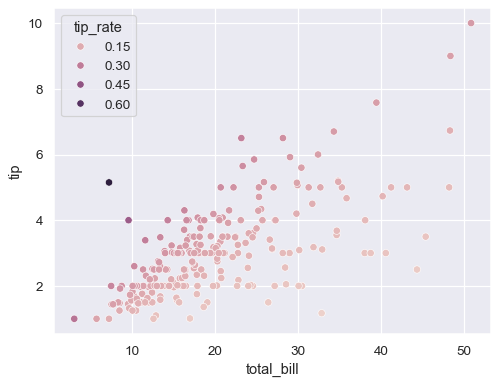A numeric variable can also be assigned to `size` to apply a semantic mapping to the areas of the points:

```sns.scatterplot(data=tips, x="total_bill", y="tip", hue="size", size="size")
```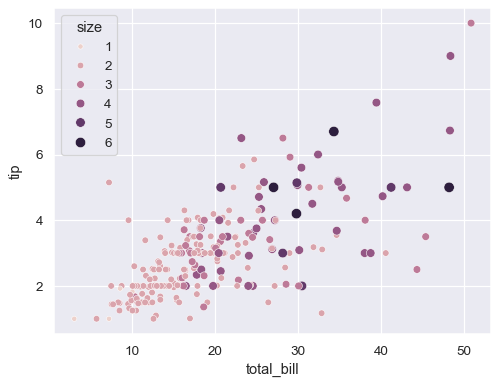Control the range of marker areas with `sizes`, and set `lengend="full"` to force every unique value to appear in the legend:

```sns.scatterplot(
data=tips, x="total_bill", y="tip", hue="size", size="size",
sizes=(20, 200), legend="full"
)
```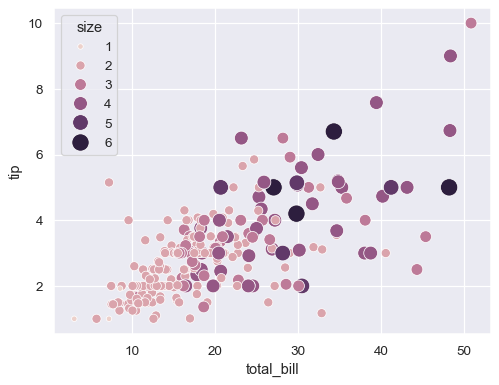Pass a tuple of values or a `matplotlib.colors.Normalize` object to `hue_norm` to control the quantitative hue mapping:

```sns.scatterplot(
data=tips, x="total_bill", y="tip", hue="size", size="size",
sizes=(20, 200), hue_norm=(0, 7), legend="full"
)
```Control the specific markers used to map the `style` variable by passing a Python list or dictionary of marker codes:

```markers = {"Lunch": "s", "Dinner": "X"}
sns.scatterplot(data=tips, x="total_bill", y="tip", style="time", markers=markers)
```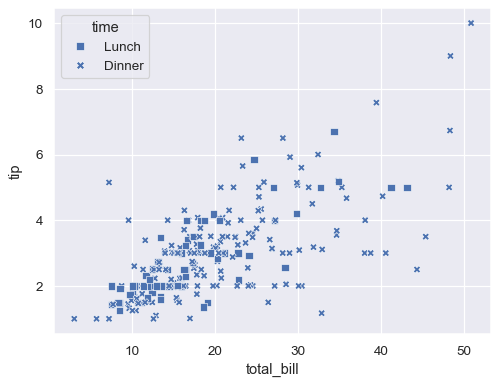Additional keyword arguments are passed to `matplotlib.axes.Axes.scatter()`, allowing you to directly set the attributes of the plot that are not semantically mapped:

```sns.scatterplot(data=tips, x="total_bill", y="tip", s=100, color=".2", marker="+")
```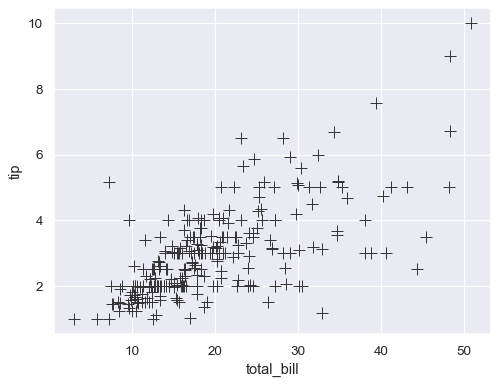The previous examples used a long-form dataset. When working with wide-form data, each column will be plotted against its index using both `hue` and `style` mapping:

```index = pd.date_range("1 1 2000", periods=100, freq="m", name="date")
data = np.random.randn(100, 4).cumsum(axis=0)
wide_df = pd.DataFrame(data, index, ["a", "b", "c", "d"])
sns.scatterplot(data=wide_df)
```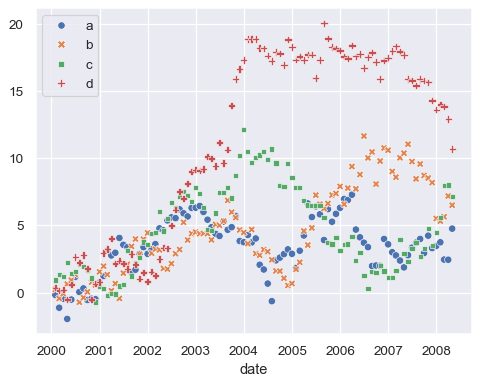Use `relplot()` to combine `scatterplot()` and `FacetGrid`. This allows grouping within additional categorical variables, and plotting them across multiple subplots.

Using `relplot()` is safer than using `FacetGrid` directly, as it ensures synchronization of the semantic mappings across facets.

```sns.relplot(
data=tips, x="total_bill", y="tip",
col="time", hue="day", style="day",
kind="scatter"
)
```